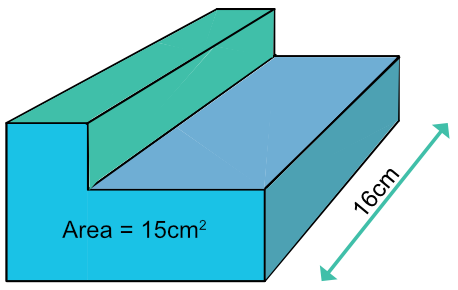Volume of a Prism

## Volume of a Prism

A prism is a 3D solid. It has the same cross-section along its entire length: if the prism is cut at right angles to its length at any point, then it has the same face on the cut sections as it does at each end.

The area of a prism is:

Volume = cross-sectional area x length## Example 1

The cross-sectional area of a prism is 20 cm2. The length of the prism is 5cm. What is the volume of the prism?

The volume of a prism is cross-sectional area x length

V = 20 x 5 = 100

 For a Prism Volume = Area x Length Substitute 345 = A xx 15 Divide both sides by 15 23 = text(Area)